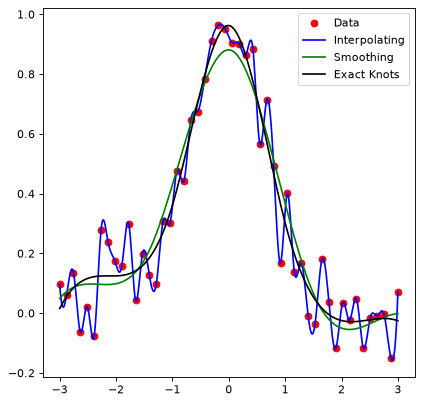# 1D Spline Models¶

Spline1D models are models which can be used to fit a piecewise polynomial to a set of data. This means that splines are closely tied to the method used to fit the spline to the data. Currently, we provide three methods for fitting splines to data:

• SplineInterpolateFitter, which fits an interpolating spline to the data. This means that the spline will exactly fit all data points.

• SplineSmoothingFitter, which fits a smoothing spline to the data. This means that the number of knots is chosen to satisfy the “smoothing condition”:

$\sum_{i} \left(w_i * (y_i - spl(x_i))\right)^{2} \leq s$
• SplineExactKnotsFitter, which fits a spline to the data using an exact set of knots. This means that the spline will use least-squares regression using the user supplied (interior) knots to find the best fit spline to the data.

import numpy as np
import matplotlib.pyplot as plt
from astropy.modeling.models import Spline1D
from astropy.modeling.fitting import (SplineInterpolateFitter,
SplineSmoothingFitter,
SplineExactKnotsFitter)

x = np.linspace(-3, 3, 50)
y = np.exp(-x**2) + 0.1 * np.random.randn(50)
xs = np.linspace(-3, 3, 1000)
t = [-1, 0, 1]
spl = Spline1D()

fitter = SplineInterpolateFitter()
spl1 = fitter(spl, x, y)

fitter = SplineSmoothingFitter()
spl2 = fitter(spl, x, y, s=0.5)

fitter = SplineExactKnotsFitter()
spl3 = fitter(spl, x, y, t=t)

plt.plot(x, y, 'ro', label="Data")
plt.plot(xs, spl1(xs), 'b-', label="Interpolating")
plt.plot(xs, spl2(xs), 'g-', label="Smoothing")
plt.plot(xs, spl3(xs), 'k-', label="Exact Knots")
plt.legend()
plt.show()


(png, svg, pdf)Note that by default, splines have degree 3. In the case of these splines, the degree - 1 is the number of derivatives that are matched by the spline across knot points. So for degree 3 splines, the value, first, and second derivatives of the spline will match across each knot point.

Warning

Splines only support integer degrees, such that 1 <= degree <= 5.